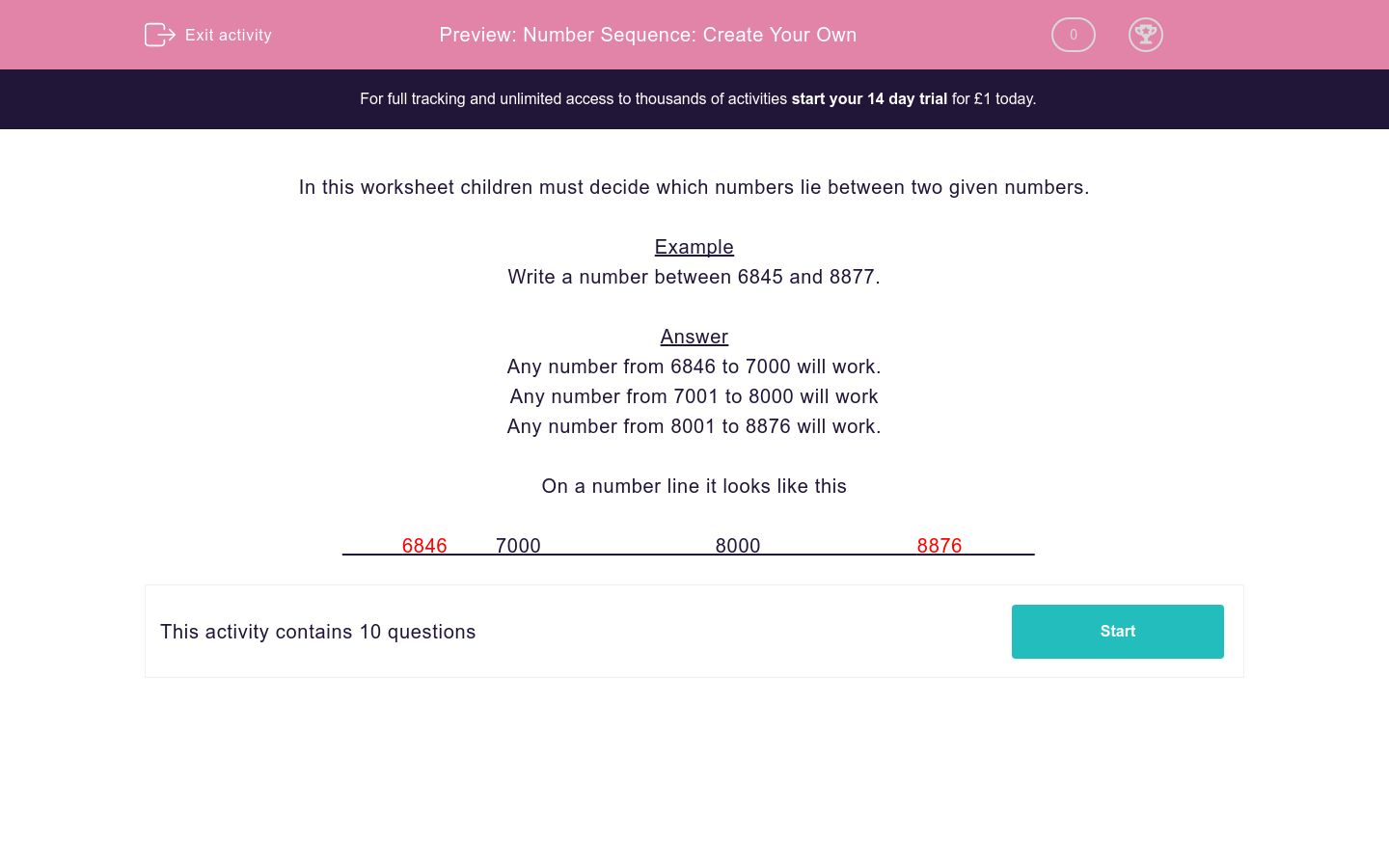# Number Sequence: Create Your Own

In this worksheet, students write an integer between the two given numbers to create their own number sequence.Key stage:  KS 2

Curriculum topic:   Maths and Numerical Reasoning

Curriculum subtopic:   Sequences

Difficulty level:### QUESTION 1 of 10

In this worksheet children must decide which numbers lie between two given numbers.

Example

Write a number between 6845 and 8877.

Any number from 6846 to 7000 will work.

Any number from 7001 to 8000 will work

Any number from 8001 to 8876 will work.

On a number line it looks like this

6846        7000                             8000                          8876

Select any numbers which are between 6541 and 7666.

1323

7659

9878

Select any numbers which are between 6758 and 9631.

4643

7659

9621

Select any numbers which are between 1306 and 7680.

4643

7659

9621

Select any numbers which are between 6558 and 8007.

3213

7999

6560

Select any numbers which are between 1187 and 2373.

3213

1188

2374

Select any numbers which are between 5002 and 9912.

6000

7000

1000

Select any numbers which are between 3438 and 3860.

3440

4653

1000

Select any numbers which are between 2565 and 6836.

3440

4653

6834

Select any numbers which are between 2378 and 2513.

1092

5235

2390

Select any numbers which are between 6650 and 6912.

6546

6666

0666

• Question 1

Select any numbers which are between 6541 and 7666.

7659
• Question 2

Select any numbers which are between 6758 and 9631.

7659
9621
• Question 3

Select any numbers which are between 1306 and 7680.

4643
7659
• Question 4

Select any numbers which are between 6558 and 8007.

7999
6560
• Question 5

Select any numbers which are between 1187 and 2373.

1188
• Question 6

Select any numbers which are between 5002 and 9912.

6000
7000
• Question 7

Select any numbers which are between 3438 and 3860.

3440
• Question 8

Select any numbers which are between 2565 and 6836.

3440
4653
6834
• Question 9

Select any numbers which are between 2378 and 2513.

2390
• Question 10

Select any numbers which are between 6650 and 6912.